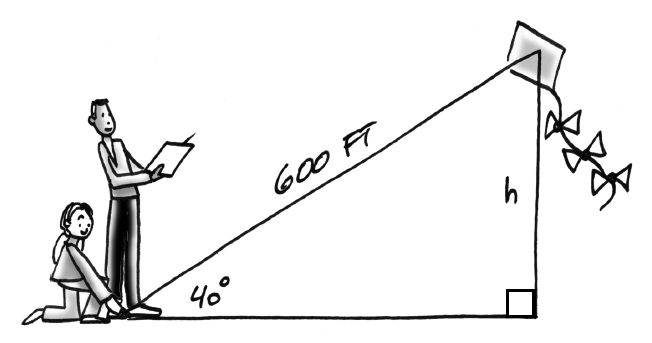Home > CCG > Chapter 6 > Lesson 6.1.5 > Problem6-52

6-52.One measurement used in judging kite-flying competitions is the size of the angle formed by the kite string and the ground. This angle can be used to find the height of the kite. Suppose the length of the string is $600$ feet and the angle at which the kite is flying measures $40º$. Calculate the height, $h$, of the kite.

What trig ratio includes the opposite side and the hypotenuse?

$\sin40º= \frac{ h }{600 }$

$h\approx385.67$ feet